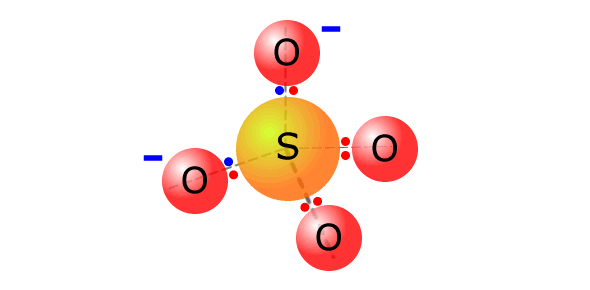# Polyatomic Ions Quiz

10 Questions | Total Attempts: 58953SettingsA polyatomic ion is seen as a molecule that has been ionized by gaining or losing electrons. In a polyatomic ion, the group of covalently bonded atoms carries a net charge because the total number of electrons in the molecule is not equal to the total number of protons in the molecule. Take up the quiz below to see how much you understood about the ions.

• 1.
What is the difference between sulfate and sulfite?
• A.

There is no difference, they are the same thing.

• B.

Sulfate has one more oxygen than sulfite.

• C.

Sulfate has a positive charge while sulfite has a negative charge.

• D.

Sulfite has one less sulfur than sulfate.

• E.

Sulfate goes to the third power, while sulfite goes to the second.

• 2.
What is the name of this chemical? NH4+
• A.

Ammonium

• B.

Nitrite

• C.

Nitrate

• D.

Hydroxide

• E.

Acetate

• 3.
What is the name of this chemical? CrO42-
• A.

Phosphate

• B.

Carbonate

• C.

Chromate

• D.

Sulfite

• E.

Sulfate

• 4.
What is NO2-
• 5.
Acetate can be shown as C2H3O2- or CH3COO-.
• A.

True

• B.

False

• 6.
What is CO32- called?
• A.

Chromate

• B.

Phosphate

• C.

Nitrite

• D.

Carbonate

• E.

Sulfate

• 7.
OH- can be described as which polyatomic ion?
• A.

Hydroxite

• B.

Phosphate

• C.

Hydroxide

• D.

Helium

• E.

Deoxyribose

• 8.
Is NO3- nitrite or nitrate?
• 9.
What does the ion phosphate contain?
• A.

1 phosphorus, 4 oxygen, to the third power negative

• B.

1 phosphorus, 4 oxygen, to the second power negative

• C.

2 phosphorus, 4 oxygen, to the third power negative

• D.

1 phosphorus, 5 oxygen, to the second power negative

• E.

2 phosphorus, 4 oxygen, to the third power negative

• 10.
Does SO32- represent sulfate or sulfite?
• A.

Sulfite

• B.

Sulfate

• C.

Both

• D.

Neither

Related Topics